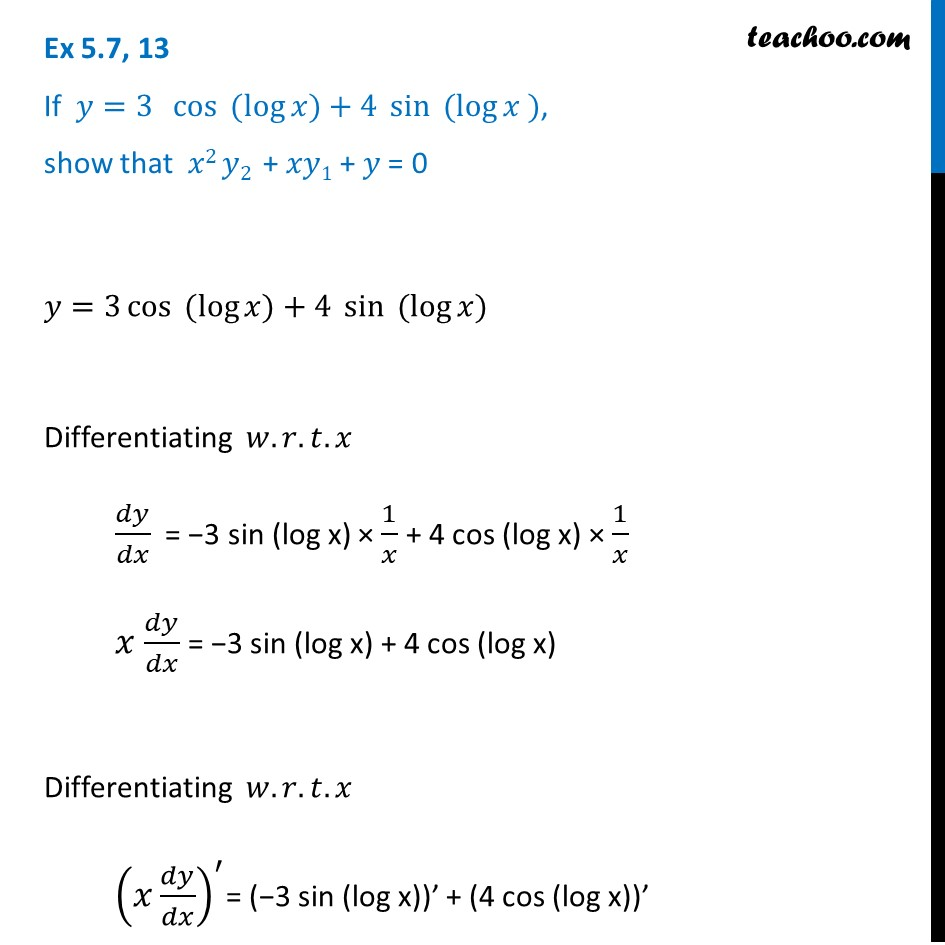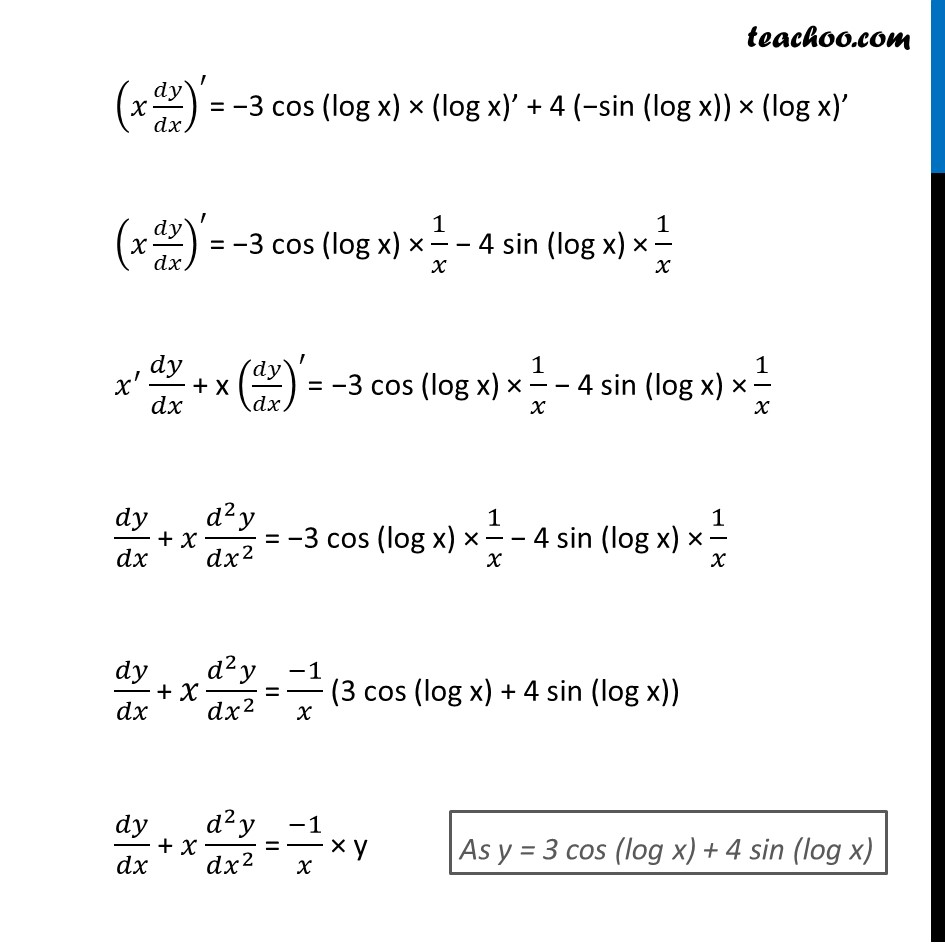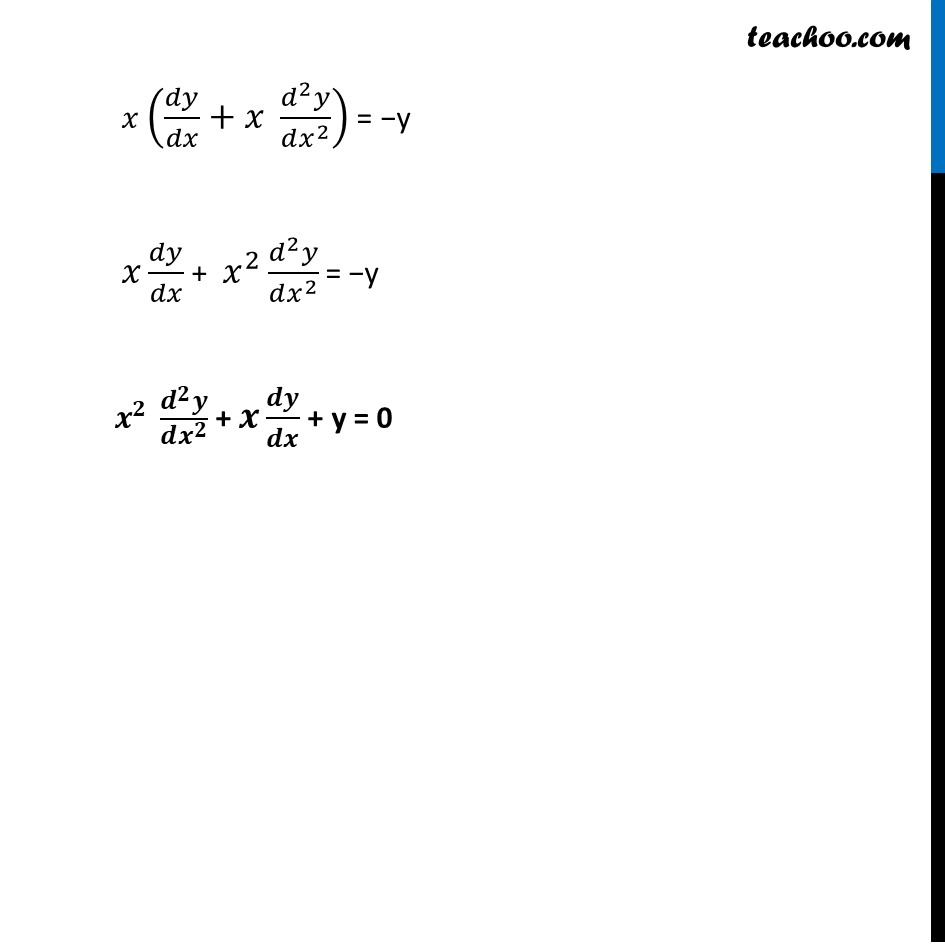Finding second order derivatives- Implicit form

Chapter 5 Class 12 Continuity and Differentiability
Concept wiseLearn in your speed, with individual attention - Teachoo Maths 1-on-1 Class

### Transcript

Ex 5.7, 13 If 𝑦=3 cos⁡〖 (log⁡〖𝑥)+4 〖 sin〗⁡〖 (log⁡〖𝑥 )〗 〗 〗 〗, show that 𝑥2 𝑦2 + 𝑥𝑦1 + 𝑦 = 0 𝑦=3 cos⁡〖 (log⁡〖𝑥)+4 〖 sin〗⁡〖 (log⁡〖𝑥)〗 〗 〗 〗 Differentiating 𝑤.𝑟.𝑡.𝑥 𝑑𝑦/𝑑𝑥 = −3 sin (log x) × 1/𝑥 + 4 cos (log x) × 1/𝑥 𝑥 𝑑𝑦/𝑑𝑥 = −3 sin (log x) + 4 cos (log x) Differentiating 𝑤.𝑟.𝑡.𝑥 (𝑥 𝑑𝑦/𝑑𝑥)^′= (−3 sin (log x))’ + (4 cos (log x))’ (𝑥 𝑑𝑦/𝑑𝑥)^′= −3 cos (log x) × (log x)’ + 4 (−sin (log x)) × (log x)’ (𝑥 𝑑𝑦/𝑑𝑥)^′= −3 cos (log x) × 1/𝑥 − 4 sin (log x) × 1/𝑥 𝑥^′ 𝑑𝑦/𝑑𝑥 + x (𝑑𝑦/𝑑𝑥)^′= −3 cos (log x) × 1/𝑥 − 4 sin (log x) × 1/𝑥 𝑑𝑦/𝑑𝑥 + 𝑥 (𝑑^2 𝑦)/(𝑑𝑥^2 ) = −3 cos (log x) × 1/𝑥 − 4 sin (log x) × 1/𝑥 𝑑𝑦/𝑑𝑥 "+" 𝑥 (𝑑^2 𝑦)/(𝑑𝑥^2 ) = (−1)/𝑥 (3 cos (log x) + 4 sin (log x)) 𝑑𝑦/𝑑𝑥 + 𝑥 (𝑑^2 𝑦)/(𝑑𝑥^2 ) = (−1)/𝑥 × y As y = 3 cos (log x) + 4 sin (log x) 𝑥 (𝑑𝑦/𝑑𝑥+𝑥 (𝑑^2 𝑦)/(𝑑𝑥^2 )) = −y 𝑥 𝑑𝑦/𝑑𝑥 + 𝑥^2 (𝑑^2 𝑦)/(𝑑𝑥^2 ) = −y 𝒙^𝟐 (𝒅^𝟐 𝒚)/(𝒅𝒙^𝟐 ) + 𝒙 𝒅𝒚/𝒅𝒙 + y = 0